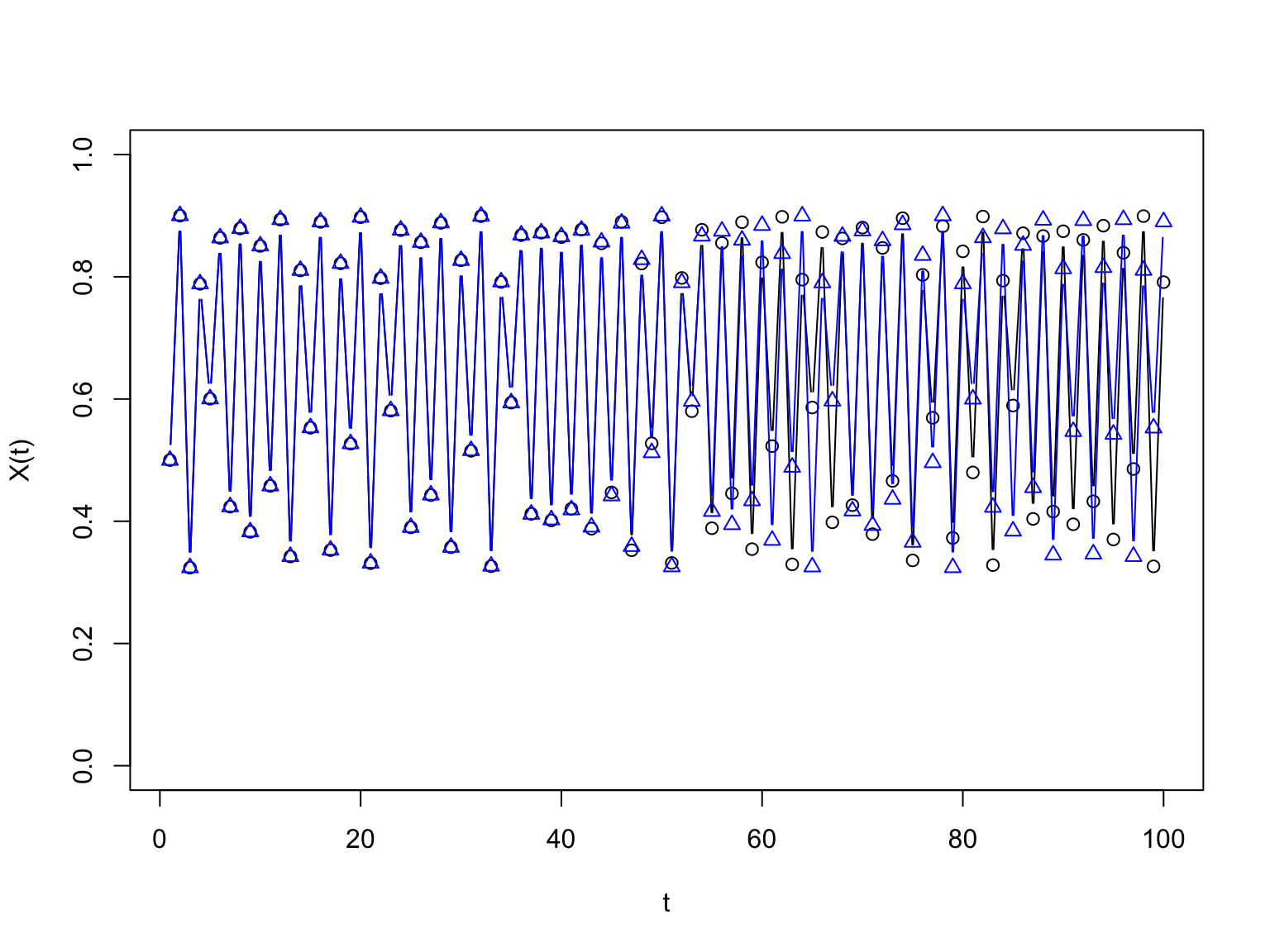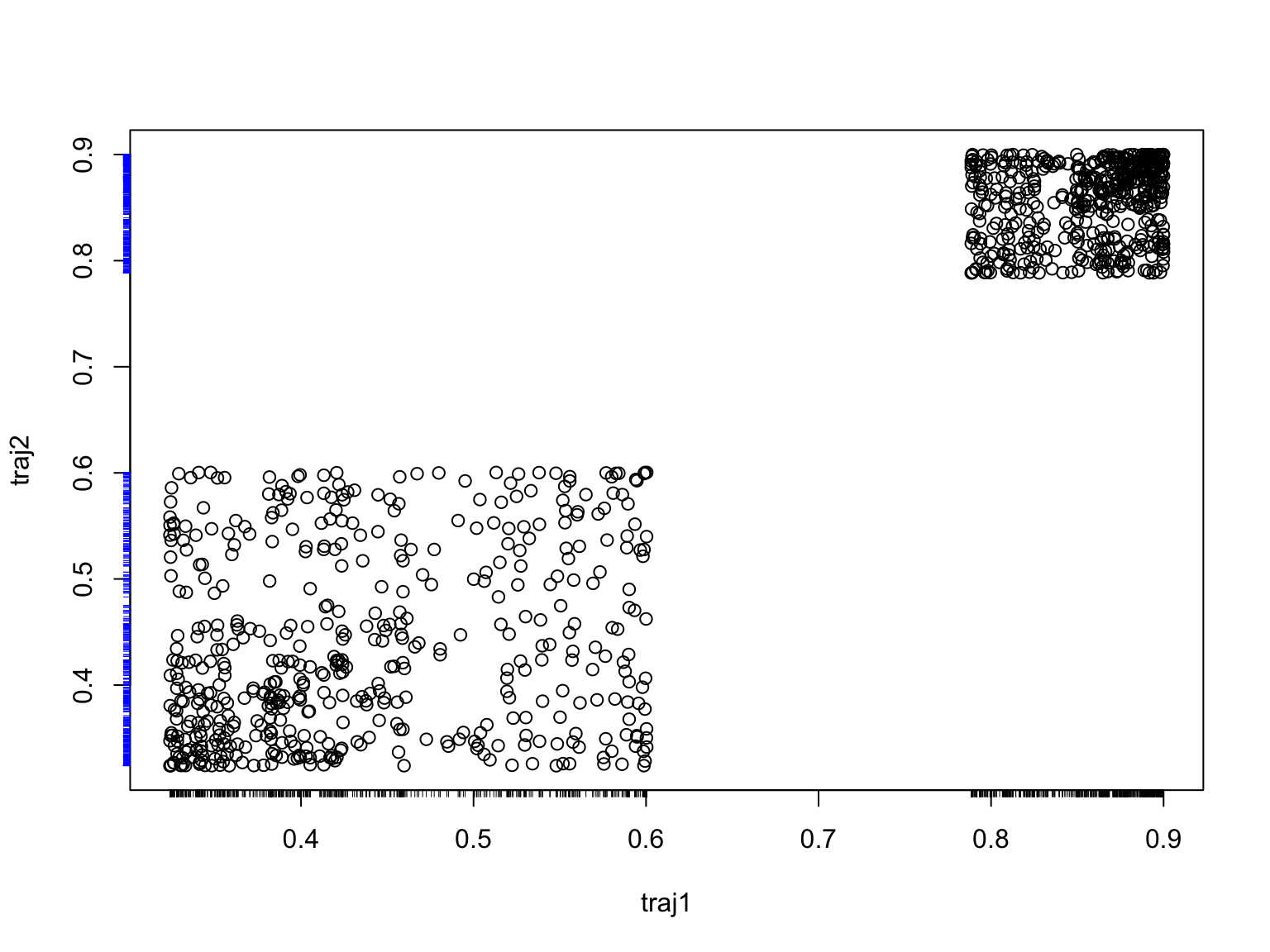Simulation for Inference II — Matching Simulations to Data

27 October 2020 (Lecture 17)

$\newcommand{\Expect}{\mathbb{E}\left[ #1 \right]} \newcommand{\Prob}{\mathbb{P}\left[ #1 \right]} \newcommand{\Probwrt}{\mathbb{P}_{#1}\left( #2 \right)} \newcommand{\Var}{\mathrm{Var}\left[ #1 \right]} \newcommand{\Cov}{\mathrm{Cov}\left[ #1 \right]} \newcommand{\SampleVar}{\widehat{\mathrm{Var}}`left[ #1 \right]} \newcommand{\Expectwrt}{\mathbb{E}_{#1}\left[ #2 \right]} \DeclareMathOperator*{\argmin}{argmin} \DeclareMathOperator*{\argmax}{argmax}$

May their memory be a blessingIn our last episodes

• Monte Carlo: figuring out what a model predicts, in detail, by simulating it
• Bootstrap: getting at sampling distributions by simulating from a good approximation to the data-generating distribution
• But how do we estimate a model in the first place…?

Agenda

• Reminder about estimation in general
• The method of simulated moments
• Auxiliary models and indirect inference
• Model checking

How we estimate, in general

$\hat{\theta}_n = \argmin_{\theta}{M_n(\theta)}$

If $$M_n(\theta) \rightarrow m(\theta)$$ as $$n\rightarrow\infty$$

and $$m(\theta)$$ has a unique minimum at the true $$\theta^*$$

then (generally) $$\hat{\theta}_n \rightarrow \theta^*$$ (consistency)

If $$m$$ has a well-behaved interior minimum, $\hat{\theta}_n \approx \theta^* - (\nabla \nabla M_n(\theta^*))^{-1} \nabla M_n(\theta^*)$ and so $\Var{\hat{\theta}_n} \approx (\nabla\nabla m(\theta^*))^{-1} \Var{\nabla M_n(\theta^*)} (\nabla\nabla m(\theta^*))^{-1}$

The Progress of Statistical Methods

1. Calculate likelihood, solve explicitly for MLE
2. Can’t solve for MLE but can still write down likelihood, calculate it, and maximize numerically
3. Even calculating the likelihood is intractable
• Outstanding example: hidden or latent variables $$Y_1, Y_2, \ldots$$ plus observed $$X_1, X_2, \ldots$$

Why Finding the Likelihood Becomes Hard

• Likelihood become an integral/sum over all possible combinations of latent variables compatible with observations: $\begin{eqnarray*} \Probwrt{\theta}{X_1^n = x_1^n} & = & \int{\Probwrt{\theta}{X_1^n = x_1^n, Y_1^n=y_1^n} d y_1^n }\\ &= & \int{\Probwrt{\theta}{Y_1^n=y_1^n}\left(\prod_{i=1}^{n}{\Probwrt{\theta}{X_i=x_i|Y_1^n=y_1^n,X_1^{i-1}=x_1^{i-1}}}\right) d y_1^n} \end{eqnarray*}$
• Evaluating this sum-over-histories is, itself, a hard problem
• Exponentially hard in $$n$$ (literally)
• One approach: Expectation-Maximization algorithm, try to simultaneously estimate latent variables and parameters (Neal and Hinton 1998)
• Standard, clever, often messy
• Another: simulate!

Method of simulated moments

Assume: the data really came from our simulation model, at some true value $$\theta^*$$ of the adjustable parameters $$\theta$$

1. Pick your favorite $$q$$-dimensional vector of statistics $$B$$ (“generalized moments”)
2. Calculate from data, $$B(obs) = B(X)$$
3. Pick a staring parameter value $$\theta$$
1. simulate multiple times, say $$s$$, getting $$\tilde{X}_1, \ldots \tilde{X}_s$$
2. calculate average of $$B$$, $$\overline{B}(\theta, n, s) \equiv \frac{1}{s}\sum_{i=1}^{s}{B(\tilde{X}_i)}$$
3. For large $$n$$, $$\overline{B}(\theta, n, s) \approx \Expectwrt{\theta}{B}$$
4. Adjust $$\theta$$ so expectations are close to $$B(obs)$$

Method of simulated moments

• How this fits into the general theory: $\begin{eqnarray} M_n(\theta) & = & \| \overline{B}(\theta,n,s) - B(obs)\|^2\\ \hat{\theta}_{MSM} & = & \argmin_{\theta}{M_n(\theta)} \end{eqnarray}$
• Assume $$\overline{B}(\theta, n, S) \rightarrow b(\theta)$$ as $$n\rightarrow\infty$$
• Assume $$B(obs) \rightarrow b(\theta^*)$$ as $$n\rightarrow\infty$$
• Then $$M_n(\theta) \rightarrow m(\theta) \equiv \| b(\theta) - b(\theta^*) \|^2$$

Method of simulated moments

• Works if the expectations of $$B$$ are enough to characterize the parameter
• i.e., if the function $$\theta \mapsto b(\theta)$$ has an inverse, so $$b^{-1}$$ is well-defined
• though for optimization we’d really prefer it to have a smooth inverse
• and if the sample versions converge on the expectations
• so we’re relying on ergodicity again, at least for these moments
• Why expectations rather than medians, modes, … ?
• Basically: easier to prove convergence
• The mean is not always the most probable value!

A challenging example

• The logistic map: $X(t+1) = 4 r X(t) (1-X(t))$
• Given $$X(1)$$, this is a deterministic dynamical system
• We’ll typically chose $$X(1)$$ as uniform on $$[0,1]$$
• For small $$r$$, $$X(t) \rightarrow 0$$
• For large $$r$$, near 1, $$X(t)$$ is chaotic
• Deterministic behavior
• Sensitive dependence on initial conditions = small perturbations get amplified exponentially (at least to start with)
• Long-run behavior is ergodic (not easy to prove!)

Sensitive dependence on initial conditions

traj1 <- logistic.map.ts(1000, r=0.9, initial.cond=0.5001)
traj2 <- logistic.map.ts(1000, r=0.9, initial.cond=0.4998)
plot(1:100, traj1[1:100], ylim=c(0,1), xlab="t", ylab="X(t)", type="b")
points(1:100, traj2[1:100], pch=2, col="blue", type="b")Sensitive dependence on initial conditions

plot(traj1, traj2)
rug(x=traj1, side=1, col="black", ticksize=-0.01) # negative sizes for pointing outward
rug(x=traj2, side=2, col="blue", ticksize=-0.01)Long-run stability

par(mfrow=c(1,2))
hist(traj1)
hist(traj2)